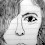Latest Articles

# Only the last term or Atyayoreva Vedic Maths

Info Post
Atyayoreva means 'Only the last term'. This is useful in solving simple equations of the following type. The type of equations are those whose numerator and denominator on the L.H.S. bearing the independent term stand in the same ratio to each other as the entire numerator and the entire denominator of the R.H.S. stand to each other. Let us have a look at the following example.
Example 1:
x2+2x +7       x+ 2
------------- =  ------
x2+3x +5       x+ 3

In the conventional method we proceed as

x2+2x +7        x+ 2
------------- =  --------
x2+3x +5        x+ 3

(x + 3) (x2 + 2x + 7) = (x + 2) (x 2+3x +5)

x3+2x 2+7x +3x 2+6x +21 = x 3+3x 2+5x +2x 2+6x +10
x3+5x 2+13x+21 = x 3+5x 2+11x+10
Cancelling the like terms on both sides ,we get

13x+21 =11x+10
13x–11x= 10 –21
2x = -11
Therefore , x= - (11/2)

Solving the same problem by Anatyayoreva,

x2 + 2x + 7      x+ 2
(L.H.S)       -------------- =  ------   (R.H.S)
x2 + 3x + 5      x+ 3

Observe the above equation where L.H.S

x2 + 2x       x ( x+ 2)      (x + 2)
---------  =   ----------   = ---------  acc. the condition in Sutra  is equal to R.H.S
x2 + 3x       x (x + 3)      (x + 3)

Now taking the L.H.S , we see that

(x + 2 )         7
---------   =   ---
(x + 3 )         5

Solving ,  5 (x +2 ) = 7 (x +3)

5x +10 =7x +21
7x -5x = 10-21
2x = -11
Therefore , x = - (11/2)

This method appears to be faster and easier than the conventional method.Also from this example, we can clearly see what "only the last term" means

Algebraic Proof:
Consider the equation
AC + D      A
---------- = -----   |--------- (1)
BC + E      B

This satisfies the condition in the sutra since
AC       A
----- = -----
BC       B

Now cross–multiply the equation (1)
B (AC + D) = A (BC + E)
BAC + BD = ABC + AE
BD = AE which gives
A      D
--- = ----    |--------(2)
B      E

i.e., the result obtained in solving equation (1) is same as the result obtained in solving equation (2)

Share:

1.Hi, friend, I;m hosting a contest on 55 Fiction. It would be really very interesting... so I'm very happy to invite you to participate... take care... "Best 55 Fictionist Contest"

2.nice. Where did you get this?

Thank You for visiting Momscribe.com !